Courses

# Spinning tops and gyroscopes Physics Notes | EduRev

## Physics : Spinning tops and gyroscopes Physics Notes | EduRev

The document Spinning tops and gyroscopes Physics Notes | EduRev is a part of the Physics Course Basic Physics for IIT JAM.
All you need of Physics at this link: Physics

Figure 23 (top) shows a wheel that is weighted in its rim to maximize its moment of inertia I and that is spinning with angular frequency ω on a horizontal axle supported at both ends. As shown, it has an angular momentum L along the x direction equal to . Now suppose the support at point P is removed, leaving the axle supported only at one end (figure 23, middle). Gravity, acting on the mass of the wheel as if it were concentrated at the centre of mass, applies a downward force on the wheel. The wheel, however, does not fall. Instead, the axle remains (nearly) horizontal but rotates in the counterclockwise direction as seen from above (figure 23, bottom). This motion is called gyroscopic precession.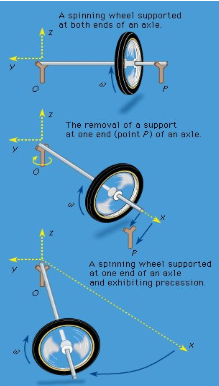Figure 23: Gyroscopic precession.

Horizontal precession occurs in this case because the gravitational force results in a torque with respect to the point of suspension, such that τ = r × F and is directed, initially, in the positive y direction. The torque causes the angular momentum L to move toward that direction according to τ = dL/dt. Because τ is perpendicular to L, it does not change the magnitude of the angular momentum, only its direction. As precession proceeds, the torque remains horizontal, and the angular momentum vector, continually redirected by the torque, executes uniform circular motion in the horizontal plane at a frequency Ω, the frequency of precession.
In reality, the motion is a bit more complicated than uniform precession in the horizontal plane. When the support at P is released, the centre of mass of the wheel initially drops slightly below the horizontal plane. This drop reduces the gravitational potential energy of the system, releasing kinetic energy for the orbital motion of the centre of mass as it precesses. It also provides a small component of L in the negative z direction, which balances the angular momentum in the positive z direction that results from the orbital motion of the centre of mass. There can be no net angular momentum in the vertical direction because there is no component of torque in that direction.
One more complication: the initial drop of the centre of mass carries it too far for a stable plane of precession, and it tends to bounce back up after overshooting. This produces an up-and-down oscillation during precession, called nutation (“nodding”). In most cases, nutation is quickly damped by friction in the bearings, leaving uniform precession.
A spinning top undergoes all the motions described above. If it is initially set spinning with a vertical axis, there will be virtually no torque, and conservation of angular momentum will keep the axis vertical for a long time. Eventually, however, friction at the point of contact will require the centre of mass to lower itself, which can only happen if the axis tilts. The spinning will also slow down, making the tilting process easier. Once the top tilts, gravity produces a horizontal torque that leads to precession of the spin axis. The subsequent motion depends on whether the point of contact is fixed or free to slip on the horizontal plane. Vast tomes have been written on the motions of tops.
A gyroscope is a device that is designed to resist changes in the direction of its axis of spin. That purpose is generally accomplished by maximizing its moment of inertia about the spin axis and by spinning it at the maximum practical frequency. Each of these considerations has the effect of maximizing the magnitude of the angular momentum, thus requiring a larger torque to change its direction. It is quite generally true that the torque τ, the angular momentum L, and the precession frequency Ω(defined as a vector along the precession axis in the direction given by the right-hand rule) are related by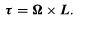(90)

Equation (90), illustrated in Figure 24, is called the gyroscope equation.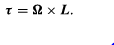(90)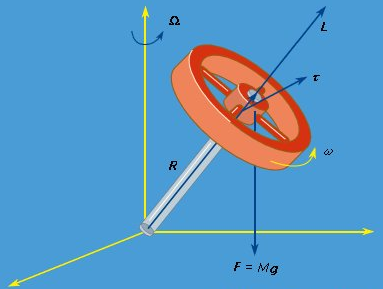Figure: A gyroscope.

Gyroscopes are used for a variety of purposes, including navigation. Use of gyroscopes for this purpose is called inertial guidance. The gyroscope is suspended as nearly as possible at its centre of mass, so that gravity does not apply a torque that causes it to precess. The gyroscope tends therefore to point in a constant direction in space, allowing the orientation of the vehicle to be accurately maintained.
One further application of the gyroscope principle may be seen in the precession of the equinoxes. The Earth is a kind of gyroscope, spinning on its axis once each day. The Sun would apply no torque to the Earth if the Earth were perfectly spherical, but it is not. The Earth bulges slightly at the Equator. As indicated in Figure 25, the effect of the Sun’s gravity on the near bulge (larger than it is on the far bulge) results in a net torque about the centre of the Earth. When the Earth is on the other side of the Sun, the net torque remains in the same direction. The torque is small but persistent. It causes the axis of the Earth to precess, about one revolution every 25,800 years.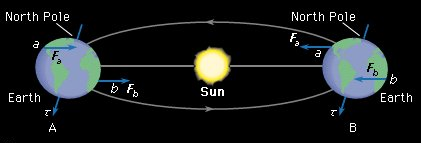Figure 25: Forces acting on equatorial bulges in (A) the summer and (B) the winter cause the axis of the Earth to precess

As seen from the Earth, the Sun passes through the plane of the Equator twice each year. These points are called the equinoxes, and on the days of the equinoxes the hours of daylight and night are equal. From antiquity it has been known that the point in the sky where the Sun intersects the plane of the Equator is not the same each year but rather drifts very slowly to the west. This ancient observation, first explained by Newton, is due to the precession of the Earth’s axis. It is called the precession of the equinoxes.

Offer running on EduRev: Apply code STAYHOME200 to get INR 200 off on our premium plan EduRev Infinity!

,

,

,

,

,

,

,

,

,

,

,

,

,

,

,

,

,

,

,

,

,

;